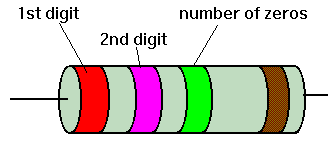Home > Electronics Tutorials > Components Tutorial > Resistors Color/Colour Code Tutorial

# Resistance/Resistors Color Codes Tutorial

Ignore the colour of the resistor body. Most resistors have three coloured bands close together at one end and one single band at the other. The three adjacent band give the resistor value.

The band nearest the wire lead gives the value of the first digit. e.g Brown = 1.

The next band gives the value of the next digit e.g. red  = 2.

The third band gives the number of zeros which follows the two digits.e.g. orange = 3 zeros = 000.

Therefore a resistor with brown, red, orange bands would have a value of 12000 ohms.This resistor has a value of 2,700,000 ohms.

A green blue black resistor would be 56 ohms. (black indicates that there are no zeros).

Black = 0
Brown = 1
Red = 2
Orange = 3
Yellow = 4
Green = 5
Blue = 6
Violet = 7
Grey = 8
White = 9

If the third band is silver then divide the value of the first two digits by 100, if gold divide by 10.
e.g. red violet gold is 2.7 ohms.

The fourth band indicates the tolerance.
e.g. brown indicates plus or minus 1%.
a 100 ohm 1% resistor can be in value between 99 ohms and 101 ohms.

brown 1%
red 2%
gold 5%
silver 10%
none 20%

If there is a fifth pink band this indicates a high stability resistor.